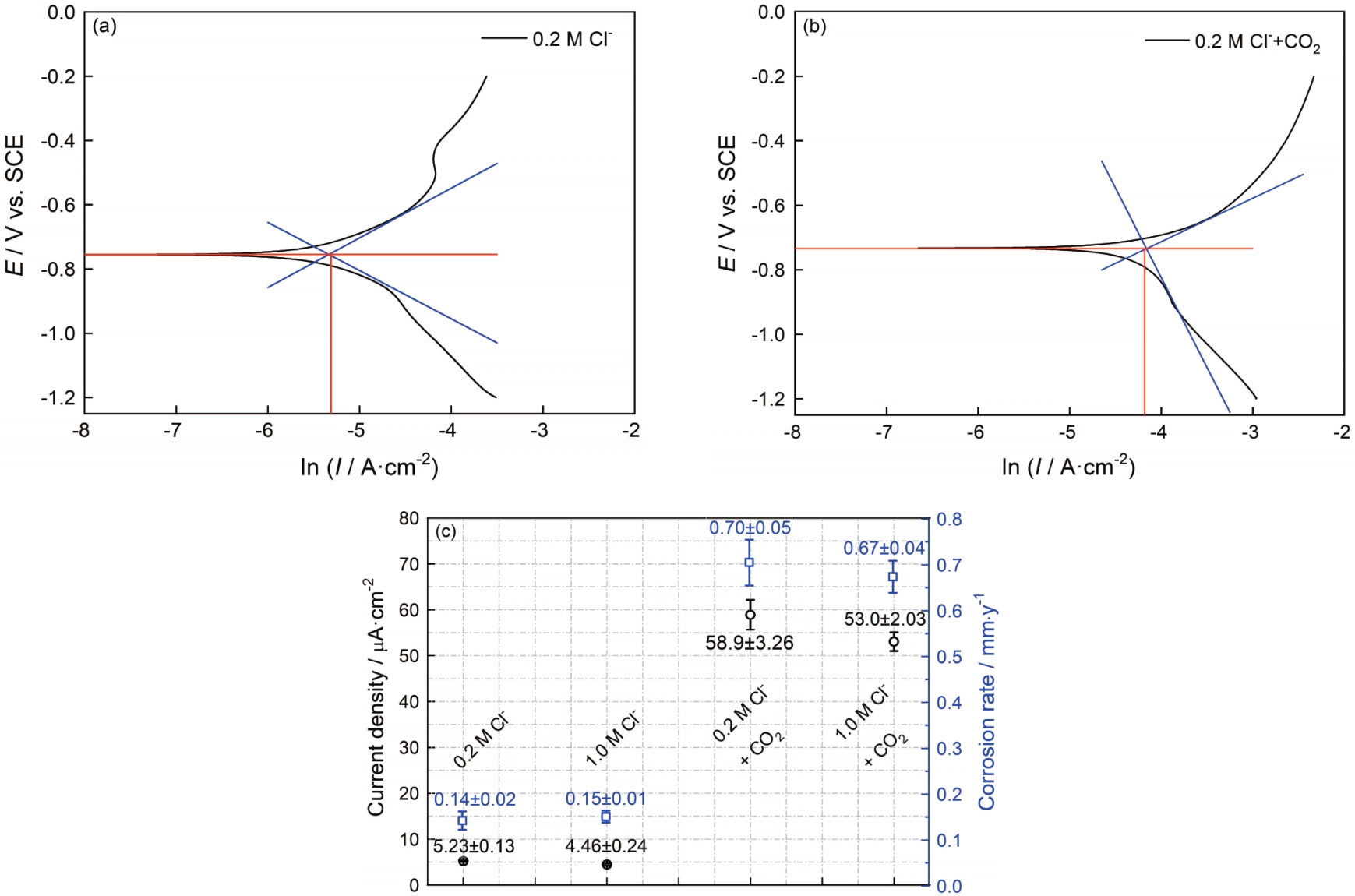# 代码逻辑不清晰，让你小白也能看懂

@数字讯号处理大作业——基于matlabR2019a的男声变女声资料汇总与代码

``````clc
%source为男声音频
%sound(y1,Fs);  %播放文件
%设计巴特沃斯低通滤波器  此处加滤波器会使声音变模糊，似乎很重要的高频分量被丢掉了
%Wc1=2*1000/Fs;                                          %男声截止频率 1000Hz
%[b,a]=butter(4,Wc1);
%y1=filter(b,a,y1);
Y1 = fft(y1);%Fast Fourier transform
sigLength=length(y1);%Length of largest array dimension
f=Fs*(1:sigLength)/sigLength;
absY=abs(Y1);%Absolute value and complex magnitude
figure('Name','Measured Data','NumberTitle','off');
%Create figure window
%Specify the Name property again, but this time, set the NumberTitle property to 'off'.
%The resulting title does not include the figure number.
%绘制男声频域
subplot(2,2,1);plot(f,absY);xlabel('Frequency(Hz)男声频域');grid on
%绘制男声时域
t=(0:sigLength-1)/Fs;%t = 1 / f
subplot(2,2,2);plot(t,y1);xlabel('Time(s)男声时域');grid on
%频谱搬移（时域相移，频域调制）
Y2 = fft(y1 .* exp(1000/(2 * pi) * 1i .* t'));
%绘制女声频域
absY2=abs(Y2);
subplot(2,2,3);plot(f,absY2);xlabel('Frequency(Hz)女声频域');grid on
%绘制女声时域
y2=ifft(Y2);
subplot(2,2,4);plot(t,y2);xlabel('Time(s)女声时域');grid on
y2=abs(y2);%audiowrite必须输入为实数
%失败的滤波器尝试
%y3 = filter(BPF.LPF_60M,1,y2);
%能调通但是效果不好的滤波器尝试
y3=highp(y2,400,500,0.0001,0.02,Fs);
audiowrite('transform30.wav',y3,Fs)
%transform30为生成的文件，它生成的地址不是很确定，会发生变化，
%我还没搞明白，以下是它出现的两个位置：
%C:\Users\honor\Documents\MATLAB\Examples\R2019a\matlab\WriteanAudioFileExample
%E:\…\dsp_greatassignment\try

``````

``````function y=highp(x,f1,f2,rp,rs,Fs)
%高通滤波,该代码鲁棒性很差，试试你就晓得了
%也可能是我不会用
%通带或阻带的截止频率的选取范围是不能超过采样率的一半
%即，f1,f3的值都要小于 Fs/2
%x:需要带通滤波的序列
% f 1：通带截止频率
% f 2：阻带截止频率
%rp：边带区衰减DB数设置
%rs：截止区衰减DB数设置
%FS：序列x的采样频率
wp=2*pi*f1/Fs;
ws=2*pi*f2/Fs;
% 设计切比雪夫滤波器；
[n,wn]=cheb1ord(wp/pi,ws/pi,rp,rs);
[bz1,az1]=cheby1(n,rp,wp/pi,'high');
%查看设计滤波器的曲线
[h,w]=freqz(bz1,az1,256,Fs);
h=20*log10(abs(h));
figure('Name','highpass filter','NumberTitle','off');
plot(w,h);title('所设计滤波器的通带曲线');grid on;
y=filter(bz1,az1,x);
end
``````fdatool和FIRMegaCore使用方法的问题